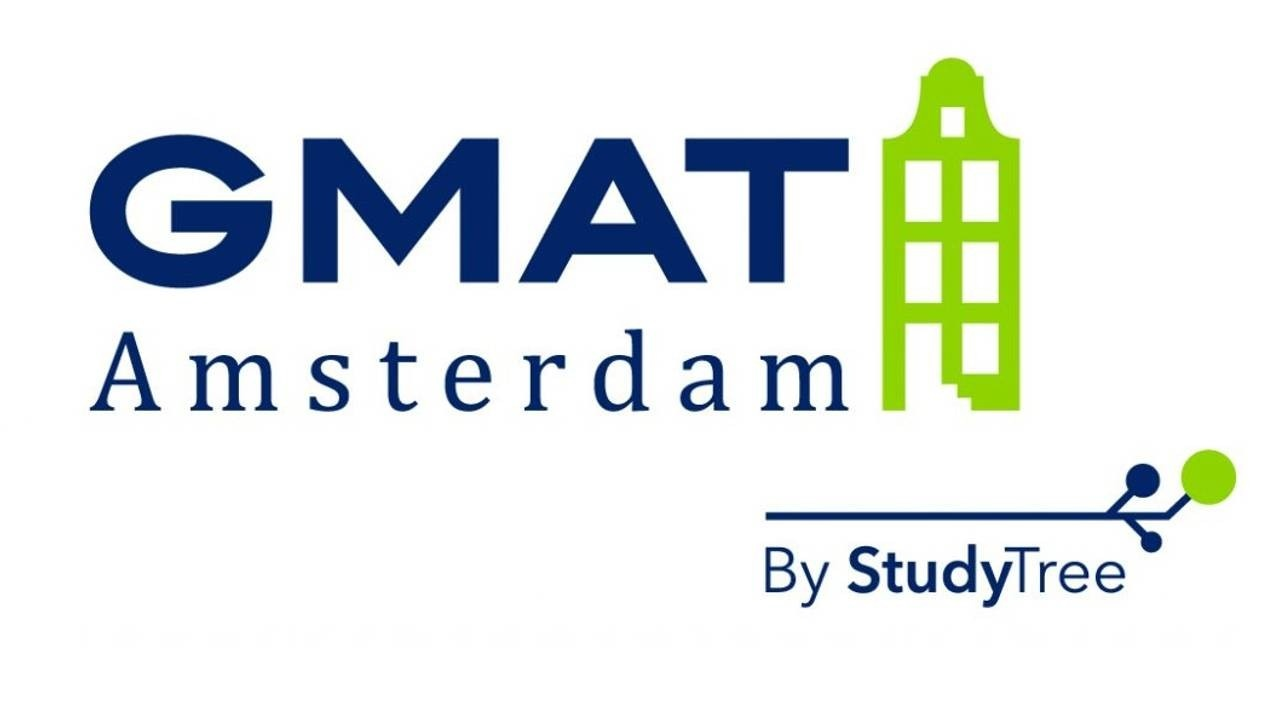>

# BASIC MATH COURSE

## Basic math course

The Basic Math course is designed to improve the mathematical skills of test takers with a weaker quantitative background. During the GMAT, you will not be allowed to use a calculator. This means you will have to do all calculations by hand.

If it has been a while since you took your last math classes or you just want to make sure your skills are sufficient before starting the Quantitative course, then this is the perfect course for you. Basic Math is a fully digital course, offered on our online platform. It is accessible 24/7 to minimize the loss of time before starting the Quantitative course.

## Our courses

We offer our courses in three attractive package deals. The courses that come with live sessions can also be bought separately. To see all details, click the button below.## Characteristics of the GMAT Basic Math course

• Development of your basic skills
• Recapitulation of the theory
• Ideal preparation for the Quantitative Course
• Fully online and accessible 24/7

### Subject 1-Fractions

##### Online portal
• 10 theory videos
• 60 practice assignments
• full recording of live session
##### Topics
• Visualisation of a fraction
• Multiply fractions
• Simplify fractions
• Multiply numbers with fractions
• Improper fraction
• Dividing fractions
• Dividing numbers and fractions
• Combining +,-,*,: fractions

### Subject 2-Exponents

##### Online portal
• 10 theory videos
• 60 practice assignments
• full recording of live session
##### Topics
• What is an exponent?
• Multiply equal base
• Divide equal base
• Multiply equal exponent
• Divide equal exponent
• Exponent of an exponent
• What is a negative power?
• What is a square root?
• Approximate square roots
• Square root as an exponent
• Simplify square root

### Subject 3-Equations

##### Online portal
• 8 theory videos
• 48 practice assignments
• full recording of live session
##### Topics
• What is a linear equation?
• Solving a 1 variable linear equations
• Linear equation with two variables
• Solving two double variable equations
• What is a quadratic equation
• A times B = 0
• Solving exponential equations

### Subject 4-Percents

##### Online portal
• 3 theory videos
• 18 practice assignments
• full recording of live session
##### Topics
• Percent vs decimal vs fraction
• Percent change
• Reverse percent trick

Basic math skills are a necessary prerequisite to solve GMAT Quantitative problems. In order to get the most out of your Quantitative Course, your basic math skills should be at the right level. Do you want to make sure that you have the right starting level to participate in this course?

To help you assess your math skills, we have created a simple self-test. You can download the test by clicking on the button below. We strongly recommend you to make the test before your start the Quantitative course.

## Course information

The Basic Math course is the ideal preparation for the Quantitative course and makes sure you will not get overwhelmed by the math while studying GMAT questions. Solid basic math skills are strongly recommended in order to increase the efficiency of your Quant preparation. We therefore recommend taking this course before the Quantitative course.

It consists of four sections with the main topics of the course: fractions, exponents, equations, and percents. The GMAT Amsterdam platform contains extensive material discussing each topic. It includes over two hours of video material, comprised of 32 clips that explain the theory in a clear and structured way. You will also find numerous practice exercises to test your knowledge, including solutions.